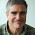Monday, October 17, 2011

Teaching PCPs to Undergrads

The last few times I've taught undergraduate theory I cover the PCP theorem. It's not complicated if you state it the right way:

PCP Theorem: For any constant α > 7/8, there is a polynomial-time computable function f mapping 3-CNFs to 3-CNFs such that for all formula φ,
• If φ is satisfiable then f(φ) is satisfiable.
• If φ is not satisfiable then every assignment to f(φ) satisfies at most an α-fraction of the clauses.
I point out to the class you can satisfy 7/8 of the clauses by just choosing a random assignment.

Also I show how the PCP theorem gives some (weak) approximation bounds for Clique. For each variable in each clause of f(φ) create a node of a graph and connect two nodes as long as they aren't in the same clause or connect a node representing a variable with one representing its negation. That gets you an 8/7-ε approximation lower bound for clique. I give showing a constant approximation lower bound for Vertex cover as a homework assignment.

I don't even try to give an idea of the proof of the PCP theorem. I just say it would take an entire graduate class to cover the proof in its full detail. That's probably a lie, one of our ten-week quarter-long classes is not enough time to prove the very strong version of the PCP theorem stated above.

1.In all seriousness: would it really not be possible to give the proof in a graduate seminar? Assuming the students had taken a graduate complexity class beforehand, I think covering the proof, in full detail, could be done in < 10 1-hour lectures.

Moreover, there have already been several classes devoted to the PCP theorem. =)

2.Lance, do you explain what the above statement has to do with the checking of proofs? (I'm assuming you tell your students what PCP stands for.)

Amit C

3.The assignment is the proof you can check with three queries by picking a random clause. I briefly mention this in class but it's the application to approximation that is more important.

4.Whats all this about Taking PCPs with undergraduates. Isn't the drug problem bad enough
without us adding to it. OH, you mean
Prob. Checkable Proofs! That's entirely different!
Never Mind.

Emily Litella

5.I once did a similar lecture many years ago for my mathematics department's undergraduate colloquium (50 minutes). Discussed the NP completeness of approximation for SAT, and related it to proof checking. No proofs of course! I did not relate it to other problems like Clique or Vertex Cover though.

This was back when I still used handwritten slides. I doubt I still have them.